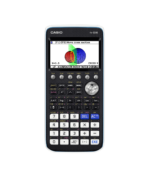Differentiation Archives - Casio Calculators

# Differentiation

Displaying Resources

FilterHow to Video
fx-CG50 A-Level IB
Calculus in Mechanics – Derivatives

How to analyse the position equation of a particle to find average, instant and ...How to Video
fx-CG50 A-Level IB
Calculus in Mechanics – Derivative Functions and Integration

How to plot and analyse the relationship between the derivative functions of the...How to Video
fx-CG50 A-Level
Tangents and Normals – Parametric Functions

How to sketch the tangent and normal to a curve in parametric form. Includes fin...How to Video
fx-CG50 A-Level IB
Tangents and Normals – Cartesian Functions

How to sketch the tangent and normal to a curve in Cartesian form. Includes find...How to Video
fx-CG50 A-Level GCSE IB
Table – Calculating Values

How to calculate a table of values for a function. Change the step interval and ...How to Video
fx-CG50 A-Level IB
Derivatives – Evaluation and Graphing

How to numerically evaluate first and second derivatives of a cartesian function...How to Video
A-Level
Calculus

How to integrate a function between limits to find the definite integral. Differ...Teach Leaflet
fx-CG50 A-Level IB
Tangents and Normals

This resource introduces the concept of a tangent and a normal to a curve and ex...Teach Leaflet
fx-CG50 IB A-Level
Derivative of Trigonometric Functions

The investigation looks at the gradient of tangents to each of the basic trigono...Teach Leaflet
fx-CG50 A-Level IB
Differentiation from First Principles

The investigation leads learners to calculate the gradient of chords from a fixe...Teach Leaflet
fx-CG50 A-Level IB

This investigation leads learners to use the calculator generated values for the...Teach Leaflet
fx-CG50 A-Level IB
Introduction to the Exponential Function

This activity looks at the shape of the graphs y = ax and examines the link betw...How to Leaflet
fx-CG50 A-Level GCSE IB
Table – Calculating Values (Worksheet)

Worksheet to accompany the video on how to calculate a table of values for a fun...How to Leaflet
fx-CG50 A-Level
Derivatives – Evaluation and Graphing (Worksheet)

Worksheet to accompany the video on how to numerically evaluate first and second...Teach Leaflet
fx-CG20 A-Level
Exploring Parametric Equations

This activity introduces parametric equations and their graphs. Explore how to c...